# JP6485059B2 - Power system stabilization device and control method - Google Patents

## Info

Publication number
JP6485059B2
JP6485059B2 JP2015009237A JP2015009237A JP6485059B2 JP 6485059 B2 JP6485059 B2 JP 6485059B2 JP 2015009237 A JP2015009237 A JP 2015009237A JP 2015009237 A JP2015009237 A JP 2015009237A JP 6485059 B2 JP6485059 B2 JP 6485059B2
Authority
JP
Japan
Prior art keywords
value
output
input
calculation unit
stabilizing
Prior art date
Legal status (The legal status is an assumption and is not a legal conclusion. Google has not performed a legal analysis and makes no representation as to the accuracy of the status listed.)
Active
Application number
JP2015009237A
Other languages
Japanese (ja)
Other versions
JP2016135041A (en
Inventor

Original Assignee

Priority date (The priority date is an assumption and is not a legal conclusion. Google has not performed a legal analysis and makes no representation as to the accuracy of the date listed.)
Filing date
Publication date
Application filed by 株式会社明電舎 filed Critical 株式会社明電舎
Priority to JP2015009237A priority Critical patent/JP6485059B2/en
Publication of JP2016135041A publication Critical patent/JP2016135041A/en
Application granted granted Critical
Publication of JP6485059B2 publication Critical patent/JP6485059B2/en
Active legal-status Critical Current
Anticipated expiration legal-status Critical

• 230000000087 stabilizing Effects 0.000 claims description 42
• 230000000116 mitigating Effects 0.000 claims description 30
• 238000010248 power generation Methods 0.000 claims description 24
• 239000003990 capacitor Substances 0.000 claims description 20
• 229920003258 poly(methylsilmethylene) Polymers 0.000 claims description 19
• 238000010521 absorption reactions Methods 0.000 claims description 16
• 239000010410 layers Substances 0.000 claims description 16
• 238000006243 chemical reactions Methods 0.000 claims description 8
• 239000003381 stabilizers Substances 0.000 claims description 6
• 238000010586 diagrams Methods 0.000 description 12
• 238000000034 methods Methods 0.000 description 5
• 238000009738 saturating Methods 0.000 description 3
• 241001442055 Vipera berus Species 0.000 description 1
• 239000002253 acids Substances 0.000 description 1
• 230000005540 biological transmission Effects 0.000 description 1
• 230000003247 decreasing Effects 0.000 description 1
• 238000005247 gettering Methods 0.000 description 1
• 230000035943 smell Effects 0.000 description 1

## Images

•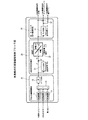•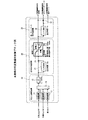•••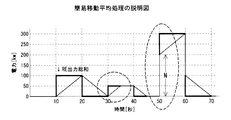•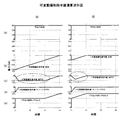••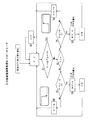•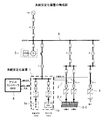•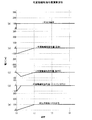## Classifications

• YGENERAL TAGGING OF NEW TECHNOLOGICAL DEVELOPMENTS; GENERAL TAGGING OF CROSS-SECTIONAL TECHNOLOGIES SPANNING OVER SEVERAL SECTIONS OF THE IPC; TECHNICAL SUBJECTS COVERED BY FORMER USPC CROSS-REFERENCE ART COLLECTIONS [XRACs] AND DIGESTS
• Y02TECHNOLOGIES OR APPLICATIONS FOR MITIGATION OR ADAPTATION AGAINST CLIMATE CHANGE
• Y02EREDUCTION OF GREENHOUSE GAS [GHG] EMISSIONS, RELATED TO ENERGY GENERATION, TRANSMISSION OR DISTRIBUTION
• Y02E70/00Other energy conversion or management systems reducing GHG emissions
• Y02E70/30Systems combining energy storage with energy generation of non-fossil origin

## Description

The present invention relates to a power system stabilization device and a control method thereof, and in particular, when a system stabilization device including a plurality of PCSs (Power Conversion Systems) having different discharge characteristics is installed in a distribution system, or a PCS installed. The present invention relates to a system stabilizing device and its control method when the total renewable energy output is larger than the capacity of the system.

When a plurality of distributed power sources are connected to the power system, a coordinated operation of the distributed power sources is performed. At that time, for example, as shown in Patent Document 1, a system stabilizing device composed of two PCSs having different discharge characteristics is installed. In order to stabilize the system, it is known.
FIG. 9 shows an example of a power distribution system in which a system stabilization device is installed. The system stabilization device 1 is composed of two systems of a lead battery system 1a and an electric double layer capacitor system 1b having different discharge characteristics. ing. 2 is a cogeneration system, 3 is a solar power generation device, 4 is a wind power generation device, and these power generation devices are arranged in parallel via a distribution line (power receiving point) 5 connected to a power transmission system. 6 is a grid controller.

In FIG. 9, since the solar power generation devices 3-1 and 3-2 and the wind power generation device 4 have power generation facilities for three types of renewable energy (hereinafter referred to as RE (Re: Renewable energy)), the weather and wind speed The generated power fluctuates greatly due to factors such as This generated power is detected, each detected value is input to the grid controller 6 to calculate a control command for the system stabilizing device 1, and the system stabilizing device 1 receives the power receiving point based on the calculated control command. It operates to mitigate power fluctuations (hereinafter referred to as RE fluctuation mitigation).

Japanese Patent No. 5141864

In the following, a case where the rated maximum output (discharge) and maximum absorption (charge) capacities of the lead battery system 1a and the electric double layer capacitor system 1b are both 100 kW will be described for simplification of description.

FIG. 10 is a waveform diagram showing a calculation example of the RE fluctuation mitigation command value to the system stabilizing device when a 100 kW fluctuation occurs. Waveform (a) is the RE output sum, waveform (b) is the entire RE fluctuation mitigation command value, Waveform (c) is the RE fluctuation relaxation command value for the PCS of the lead battery system, waveform (d) is the RE fluctuation relaxation command value for the PCS of the electric double layer capacitor system, and waveform (e) is the total RE output + PCS (power receiving point). Each power waveform.

Assuming that the total RE output indicated by (a) fluctuates from 0 to 100 kW at time t1, the total RE fluctuation relaxation command value from the total command calculation unit 20 described later is a command value in the direction of absorption as shown in (b). Is generated. Based on this command value, first, the electric double layer capacitor system shown in (d) starts the absorption operation sharply, and then the slow lead battery system starts the absorption operation as shown in (c). Even if the RE output total changes suddenly, the PCS operates separately until time t2 so that the receiving point power in (e) does not change suddenly, thereby mitigating power fluctuations.

On the other hand, depending on the system configuration, the RE output sum may be larger than the PCS capacity. FIG. 6A shows a conventional command value calculation example for mitigating RE fluctuation when the total sum of RE fluctuates from 0 to 300 kW.

Assuming that the total RE output varies from 0 to 300 kW at time t1, the entire RE fluctuation relaxation command value from the entire command calculation unit 20 generates a command value in the direction of absorption as in the waveform (b). Based on this command value, the PCS of the electric double layer capacitor system indicated by the waveform (d) performs a steep absorption operation up to the maximum capacity of 100 kW. As shown in waveform (c), the slow lead-acid battery system PCS performs normal absorption operation up to the maximum capacity of 100 kW, but when it exceeds 100 kW, the maximum capacity is exceeded. Saturates. When the command value is saturated, the RE fluctuation mitigation operation cannot be performed when the fluctuation of the RE output sum is continuously generated, and the mitigation operation of the power receiving point extends to time t4 as shown by the waveform (e). .

FIG. 7A shows the case where the total RE output is larger than the capacity of the PCS, and continuously changes from 0 to 300 kW at time t1, from 300 kW to 200 kW at time t2, and from 200 kW to 300 kW at time t3. This shows an example of command value calculation for mitigating RE fluctuation. In this example, since the PCS of the lead battery system indicated by the waveform (c) is saturated at the time t2, the fluctuation relaxation control of the receiving point power is not possible as in the part surrounded by the dotted line indicated by the waveform (e). It has become.

An object of the present invention is to provide an RE fluctuation mitigation control apparatus and a control method that prevent saturation of an RE fluctuation mitigation command value.

The present invention connects a power generation facility of renewable energy RE to a distribution system and a system stabilization device having charge / discharge characteristics, and the system stabilization device via a grid controller based on a change in power receiving point power of the distribution system. In the one that relieves rapid change of receiving point power by controlling power absorption and power generation,
The grid controller is provided with a tidal fluctuation mitigation control block,
An RE output amplitude calculation unit that outputs an RE output total value to the tidal current fluctuation mitigation control block;
Input follow-up value calculation unit for calculating the input follow-up value by inputting the RE output sum value, subtracting the input follow-up value calculated from the RE output sum value, and power absorption and power generation of the system stabilizing device based on the difference And an overall command value calculation unit having a conversion table for converting to a predetermined change slope and outputting it,
The output of the overall command value calculation unit is configured to output as an RE fluctuation mitigation command value to the system stabilizing device.

In addition, the present invention comprises a system stabilization device composed of a system stabilization device comprising a plurality of PCSs of a lead battery system and an electric double layer capacitor system having different charge / discharge characteristics,
The RE output amplitude calculation unit is configured to calculate the amplitude of the renewable energy and output the RE output sum value subjected to noise removal processing by the low-pass filter,
The output of the overall command value calculation unit is used as the RE fluctuation relaxation command value for the entire system stabilizing device, the output of the overall command value calculation unit is input to the input tracking value calculation unit, and the input tracking value is calculated to obtain the input tracking value. A lead-capacitor command that outputs a value obtained by subtracting an input follow-up value from the overall RE fluctuation relaxation command value as the RE fluctuation relaxation command value of the electric double layer capacitor system as the RE fluctuation relaxation command value of the lead battery system And an arithmetic unit.

The overall command value calculation unit of the present invention adds or subtracts a correction value N that is equal to or less than the rated processing of the system stabilizing device when the RE output total value exceeds the rating of the system stabilizing device, and thereafter The input follow-up value calculation unit calculates the input follow-up value.

Further, the present invention connects a power generation facility for renewable energy RE to a power distribution system, and a system stabilization device composed of a plurality of PCSs of a lead battery system and an electric double layer capacitor system having different charge / discharge characteristics. In a control method for controlling power absorption and power generation by a grid stabilizing device via a grid controller based on a change in point power to mitigate a rapid change in receiving point power,
The RE output total value calculated by the RE output amplitude calculation unit is input to the grid controller to the input tracking value calculation unit of the overall command value calculation unit, and the difference between the RE output total value and the output of the input tracking value calculation unit Is output as an RE fluctuation mitigation command value, when the RE output total value exceeds the rating of the system stabilizing device, a correction value N that is equal to or less than the rated processing of the system stabilizing device is added or subtracted, and then The input follow-up value calculation unit calculates the input follow-up value.

The calculation of the overall command value calculation unit according to the present invention is based on the relationship between the RE output total value and the input follow-up value, where the RE output sum value is a and the input follow-up value is a. It is characterized in that the calculation is performed based on the following formulas (1) to (4) in the magnitude relationship of a.
(1), (RE output total value a)-(input follow-up value a)> When the system stabilizer is rated,
(Input follow-up value A)-(RE output total value A) =-Rating system stabilizing device ... The input follow-up value A is abruptly changed as the correction value N so as to satisfy the equation (1).
(2), (RE output total value a) − (input follow-up value a) <when the system stabilizer is rated,
(Input follow-up value A)-(RE output summation value A) = Rating system stabilization device ...... The input follow-up value A is abruptly changed as the correction value N so as to satisfy the equation (2).
(3), (RE output total value a)> (input follow-up value a) (input follow-up value a) + (adjustment value n) Equation (3).
(4), (RE output total value a) <(input follow-up value a) (input follow-up value a)-(adjustment value n) (4)

As described above, according to the present invention, it is possible to perform the RE fluctuation mitigation operation without saturating the RE fluctuation mitigation command value even if RE fluctuations in which the power at the receiving point exceeds the rating of the system stabilizing device continuously occur. It will be.

The RE fluctuation relaxation control block diagram which shows embodiment of this invention. The RE fluctuation relaxation control block diagram which shows other embodiment of this invention. Explanatory drawing of an input tracking process. Explanatory drawing of an input tracking process. Explanatory drawing of an input tracking process. In the RE fluctuation relaxation command value calculation waveform diagram, (A) shows no command value saturation countermeasure and (B) shows a command value saturation countermeasure. In the RE fluctuation relaxation command value calculation waveform diagram, (A) shows no command value saturation countermeasure and (B) shows a command value saturation countermeasure. An input tracking value calculation flowchart. The lineblock diagram of a system stabilization device. RE fluctuation relaxation command value calculation waveform diagram.

FIG. 1 shows a schematic diagram of an RE fluctuation mitigation control block in the grid controller 6 of the present invention. The fluctuation mitigating operation of the system stabilizing device according to the present invention is performed by using each of the generated power of the renewable energy RE of the three units (solar power generation devices 3-1, 3-2, wind power generation device 4) as the RE output amplitude calculation unit 10. Are respectively input to the amplitude calculators 11, 12, and 13 to calculate the amplitude, and the adder 14 calculates the total sum of REs. The obtained RE output sum is passed through the low-pass filter 15 and subjected to noise removal processing, and is input to the overall command calculation unit 20. The overall command calculation unit 20 calculates command values for alleviating RE fluctuations for the PCS of the lead battery system 1a and the PCS of the electric double layer capacitor system 1b.

That is, the RE output sum total from the RE output amplitude calculation unit 10 is input to the input follow-up value calculation unit 21, and the input follow-up value calculation unit 21 subtracts the input follow-up value A from the RE output sum total value A to obtain the difference. Is input to the conversion table 22. In the conversion table 22, the entire RE fluctuation relaxation command value based on the charge / discharge operation state and its value is calculated based on the difference and its polarity, and output to the lead / capacitor command value calculation unit 30.
The characteristics of the conversion table 22 may be characteristics as shown in FIG.

FIG. 3 shows a subtraction mode between the RE output total value A and the input follow-up value A. FIG. 3A shows a case where the RE output sum value A> the input follow-up value A, and FIG. This is the case of the input tracking value a. Times t1 to t2 when the RE output summation value a that changes from time to time becomes large as shown in FIG. 5A or as shown in FIG. predetermined time Te between smell △ every t performs addition or calculation for subtracting the adjustment value n, the direction of the temporally constant target input following values to determine whether large or small relative to the target value of the input signal Adjust to approach the slope.

The lead / capacitor command value calculation unit 30 outputs the output of the overall command calculation unit 20 as it is as the overall RE fluctuation relaxation command value, and inputs the entire RE fluctuation relaxation command value to the input follow-up value calculation unit 31 to It outputs as RE fluctuation relaxation command value with respect to PCS of the battery system 1a. The input follow-up value from the input follow-up value calculation unit 31 is subtracted from the entire RE fluctuation mitigation command value in the subtraction unit 32, and the difference is the RE fluctuation mitigation command for the PCS of the electric double layer capacitor system 1b. Output as a value. Similar to the input follow-up value calculator 21, the input follow-up value calculator 31 determines whether it is larger or smaller than the target value of the input signal, and adjusts so as to approach the direction of the target input follow-up value with a constant slope in time. To do.

The grid controller 6 calculates the current command value for each PCS of the lead battery system 1a and the electric double layer capacitor system 1b by performing the above calculation, and the PCS of each system receives and outputs current based on the command value. Control to do.

The input follow-up value calculation unit is input separately for each of the following conditions (1) to (4) in relation to the rating of the system stabilizer and the magnitude relationship between the RE output total value A and the input follow-up value A: A function for performing a follow-up value calculation is provided, and power absorption and power generation operations as shown in FIG. 4 are performed as a system stabilization device. If the input of the input follow-up value calculation unit 21 is a constant value, the output of the calculation unit 21 outputs the same value.

(1) When the RE output sum is rapidly increasing (RE output sum value a)-(input follow-up value a)> = equipment rating (power absorption operation, charging operation to the system stabilization device),
(Input follow-up value a) − (RE output total value a) = − device rating... (Input follow-up value a) is suddenly changed as the correction value N so as to satisfy the equation (1). Subsequent calculations are based on equation (3) (operations in FIGS. 4A and 4B).

(2) When the RE output total is rapidly decreasing (RE output total value a)-(input follow-up value a) <= device rating (power generation operation, discharge operation for the system stabilization device),
(Input follow-up value a) − (RE output total value a) = equipment rating... (Input follow-up value a) is suddenly changed as a correction value N so as to satisfy the equation (2). Subsequent calculations are based on equation (4) (operations in FIGS. 4C and 4D).

(3) When (RE output total value a)> (input follow-up value a) other than (1) and (2) above,
(Input follow-up value A) + n (3) This + n value is repeatedly added every predetermined time as a new (input follow-up value a). Calculation is performed when (input follow-up value a) increases simultaneously with (RE output total value a), that is, when the input / output of the input follow-up value calculation unit 21 becomes the same value, at the same time it repeatedly outputs for each addition. Is completed (operations in FIGS. 4E and 4F).
Here, n is an adjustment value of 1 relaxation. For example, when an input tracking operation is performed in a 1 ms cycle, n = 1 ms / 10 s * 100 kW = 10 W (select a slope value that varies 100 kW in 10 seconds). .

(4) Other than the above, when (RE output total value a) <(input tracking value a),
(Input follow-up value A) -n (4) The -n value is newly subtracted every predetermined time as a new (input follow-up value a). At the same time as repeatedly outputting for each subtraction, the subtracted (input follow-up value a) becomes the same value as (RE output total value a), that is, the input / output of the input follow-up value calculation unit 21 becomes the same value. The calculation ends (the operation in FIG. 4G).
Note that no operation is performed when (RE output total value a) = (input follow-up value a).

FIG. 5 is a waveform diagram for explaining the input follow-up value processing, in which the RE output total is changed to 100 kW at the time 10 to 20 seconds, 50 kW at the time 30 to 40 seconds, and 300 kW at the time 50 to 60 seconds. In the figure, the thin line is the input follow-up value. Normally, when the input tracking process is performed, the input tracking process always changes with the same slope following the slope of the system stabilizing device at a constant 100 kW regardless of the size of the total RE output. Further, when the overall RE fluctuation relaxation command value changes to 300 kW as in the time of 50 to 60 seconds, the input follow-up value is corrected to the correction value N for 200 kW because there is no relaxation capacity of 100 kW or more of the device rating here. Change suddenly (so-called gettering) by the minute.

FIG. 6B shows an example of the calculation of the RE fluctuation relaxation command value according to the present invention, which is contrasted with the case of no command value saturation countermeasure of FIG. When the power generation changes to 300 kW, the power that can be absorbed by the system stabilizing device 1 is 100 kW. Therefore, when the input follow-up value calculation unit 21 adds 200 kW as a correction value N to the output of the input follow-up value, the power changes. At the same time, it performs an operation to continue adding the difference value after being suddenly changed relaxation adjustment value n described above in the calculation of the input tracking from (100 kW) to the same value as the RE output sum value a has become equal When complete the relaxation operation. At this time, the calculation of the correction value N is performed by the equation (1), and the PCSs of the lead battery system 1a and the electric double layer capacitor system 1b of the system stabilizing device 1 are respectively shown as waveforms (c) and (d). Power absorption operation is performed under rated conditions.

The sudden change of 200 kW with the gain is power that cannot be absorbed by the system stabilizing device 1 by more than 100 kW, so the power at the receiving point is 200 kW as shown by the waveform (e). It has become. After that, based on the formula (3), for example, as described above, the slope of 100 kW fluctuation is selected in 10 seconds, and the RE fluctuation mitigation command is terminated at time t2 without saturating the RE fluctuation mitigation command value of the lead battery system 1a. .

FIG. 7 shows a comparison waveform diagram of the conventional diagram (A) and the diagram of the present invention (B) in the case of sudden change from 300 kW to 200 kW during the RE fluctuation mitigation operation, which was different from the original operation at the sudden change. Perform the action.
In FIG. 7B, for example, it is assumed that the RE output total changes from 300 kW to 200 kW at time t2. Since it is possible to generate 100 kW of power from the system stabilizing device 1, the sudden variation for the getter is 100 kW according to equation (2), and the subsequent calculation is −n from the input follow-up value of equation (4). Subtraction is performed to select a predetermined slope. As a result, as shown by the waveform (e) in FIG. 7B, the fluctuation relaxation operation can be performed even in the case of a sudden change during the relaxation operation.

FIG. 8 shows a flowchart of the input follow-up value calculation unit 21. Step S1 is a calculation operation start condition, and it is determined whether or not the RE output total value a has changed to a predetermined power value or more. If it is within the predetermined value, it goes into a standby state in the memory as (RE output total value a = input follow-up value a) through step S2. When it is determined in step S1 that the power value has changed to a predetermined power value or more, it is determined in step S3 whether or not A> I. If YES, in S4, (A)> (device rating A). That is, it is determined whether the rating of the system stabilizing device described above and the magnitude relationship between the RE output total value A and the input follow-up value A are in the state (1). In the case of No, in S5, the 1-relaxation adjustment value n is added to the input follow-up value a to obtain a new input follow-up value a output value, and every predetermined time Δt until it becomes the same value as the RE output total value a. Repeat the operation. This state is, for example, the section from time t1 to t2 in the example of FIG.

When the state (1) is determined, N = A-A is calculated in step S6. For example, in the example of FIG. 4A, the calculation of steps S4 and S6 is performed at time t1 to calculate N for the getter, and the input follow-up value A is set as the correction value N.

On the other hand, if No in step S3, it is determined in step S7 whether or not phenomenon (2), that is, (Ai) <(device rating A). In the case of No, in step S8, the 1-relaxation adjustment value n is subtracted from the input follow-up value a to obtain a new input follow-up value a, which is repeatedly calculated every predetermined time Δt until it becomes the same value as the RE output total value a. I do. In the example of FIG. 4C, this is a section from time t1 to t2.
When the state (2) is determined, N = A + A is calculated in step S9 to calculate N for the getter, and the input follow-up value A is set as the correction value N.

In the above description, the system stabilizing device provided with a plurality of PCSs having different discharge characteristics in the distribution system has been described. In the present invention, as the system stabilizing device, either a lead battery system or an electric double layer capacitor system is used. Even if only one or a combination of both is applicable, the present invention can also be applied to a system stabilizing device in the case where the RE output sum is larger than the PCS capacity.

As described above, according to the present invention, even if RE fluctuations whose receiving point power exceeds the rating of the system stabilizer occur continuously, the RE fluctuation mitigation operation can be performed without saturating the RE fluctuation mitigation command value. is there.

DESCRIPTION OF SYMBOLS 1 ... Grid stabilization device 2 ... Cogeneration system 3 ... Solar power generation device 4 ... Wind power generation device 5 ... Distribution line (power receiving point)
6 ... Grid controller 10 ... RE output amplitude calculator 20 ... Overall command value calculator 21 ... Input tracking value calculator 22 ... Conversion table 30 ... Lead / capacitor command value calculator 31 ... Input tracking value calculator

## Claims (5)

1. Renewable energy RE power generation equipment and a grid stabilization device with charge / discharge characteristics are connected to the distribution system, and power is absorbed and distributed by the grid stabilization device via the grid controller based on changes in the distribution point power of the distribution system. In the control of the occurrence to mitigate the rapid change in receiving point power,
The grid controller is provided with a tidal fluctuation mitigation control block,
An RE output amplitude calculation unit that outputs an RE output total value to the tidal current fluctuation mitigation control block;
Input follow-up value calculation unit for calculating the input follow-up value by inputting the RE output sum value, subtracting the input follow-up value calculated from the RE output sum value, and power absorption and power generation of the system stabilizing device based on the difference And an overall command value calculation unit having a conversion table for converting to a predetermined change slope and outputting it,
The output of the overall command value calculation unit is configured to output as an RE fluctuation relaxation command value to the system stabilizing device ,
The total command value calculation unit adds or subtracts a correction value N that is equal to or less than the rated processing of the system stabilizing device when the RE output total value exceeds the rating of the system stabilizing device, and then performs the input tracking. A power system stabilization device characterized in that an input follow-up value is calculated by a value calculation unit.
2. Renewable energy RE power generation equipment and a grid stabilization device with charge / discharge characteristics are connected to the distribution system, and power is absorbed and distributed by the grid stabilization device via the grid controller based on changes in the distribution point power of the distribution system. In the control of the occurrence to mitigate the rapid change in receiving point power,
The grid controller is provided with a tidal fluctuation mitigation control block,
An RE output amplitude calculation unit that outputs an RE output total value to the tidal current fluctuation mitigation control block;
Input follow-up value calculation unit for calculating the input follow-up value by inputting the RE output sum value, subtracting the input follow-up value calculated from the RE output sum value, and power absorption and power generation of the system stabilizing device based on the difference And an overall command value calculation unit having a conversion table for converting to a predetermined change slope and outputting it,
The output of the overall command value calculation unit is configured to output as an RE fluctuation relaxation command value to the system stabilizing device ,
The system stabilizing device is composed of a system stabilizing device composed of a plurality of PCSs of a lead battery system and an electric double layer capacitor system having different charge / discharge characteristics,
The RE output amplitude calculation unit is configured to obtain the amplitude of the renewable energy RE and output the RE output total value subjected to noise removal processing by a low-pass filter,
The output of the overall command value calculation unit is used as the RE fluctuation relaxation command value of the entire system stabilizing device, the output of the overall command value calculation unit is input to the input tracking value calculation unit, and the input tracking value is calculated. Is used as the RE fluctuation relaxation command value of the lead battery system, and the value obtained by subtracting the input follow-up value from the overall RE fluctuation relaxation command value is output as the RE fluctuation relaxation command value of the electric double layer capacitor system. A power system stabilization device comprising: a command calculation unit.
3.   The total command value calculation unit adds or subtracts a correction value N that is equal to or less than the rated processing of the system stabilizing device when the RE output total value exceeds the rating of the system stabilizing device, and then performs the input tracking. The power system stabilization device according to claim 2, wherein an input follow-up value is calculated by a value calculation unit.
4. Based on the change in receiving power of the distribution system, connecting the power generation facility of renewable energy RE to the distribution system, and a system stabilization device consisting of multiple PCSs of lead battery system and electric double layer capacitor system with different charge / discharge characteristics In the control method to reduce the rapid change of receiving point power by controlling the power absorption and power generation by the grid stabilization device via the grid controller,
The RE output total value calculated by the RE output amplitude calculation unit is input to the grid controller to the input tracking value calculation unit of the overall command value calculation unit, and the difference between the RE output total value and the output of the input tracking value calculation unit Is output as an RE fluctuation mitigation command value, when the RE output total value exceeds the rating of the system stabilizing device, a correction value N that is equal to or less than the rated processing of the system stabilizing device is added or subtracted, and then An input tracking value calculation unit calculates an input tracking value, and a method for controlling a power system stabilizing device.
5. The calculation of the overall command value calculation unit is based on the relationship between the rating of the system stabilizing device and the RE output total value A and the input follow-up value A, where the RE output total value is A and the input follow-up value is A. 5. The method of controlling a power system stabilizing device according to claim 4, wherein the calculation is performed based on the following formulas (1) to (4) in the magnitude relation.
(1), (RE output total value a)-(input follow-up value a)> When the system stabilizer is rated,
(Input follow-up value A)-(RE output total value A) =-Rating system stabilizing device ... The input follow-up value A is abruptly changed as the correction value N so as to satisfy the equation (1).
(2), (RE output total value a) − (input follow-up value a) <when the system stabilizer is rated,
(Input follow-up value A)-(RE output summation value A) = Rating system stabilization device ...... The input follow-up value A is abruptly changed as the correction value N so as to satisfy the equation (2).
(3), (RE output total value a)> (input follow-up value a) (input follow-up value a) + (adjustment value n) Equation (3).
(4), (RE output total value a) <(input follow-up value a) (input follow-up value a)-(adjustment value n) (4)
JP2015009237A 2015-01-21 2015-01-21 Power system stabilization device and control method Active JP6485059B2 (en)

## Priority Applications (1)

Application Number Priority Date Filing Date Title
JP2015009237A JP6485059B2 (en) 2015-01-21 2015-01-21 Power system stabilization device and control method

## Applications Claiming Priority (1)

Application Number Priority Date Filing Date Title
JP2015009237A JP6485059B2 (en) 2015-01-21 2015-01-21 Power system stabilization device and control method

## Publications (2)

Publication Number Publication Date
JP2016135041A JP2016135041A (en) 2016-07-25
JP6485059B2 true JP6485059B2 (en) 2019-03-20

# Family

## Family Applications (1)

Application Number Title Priority Date Filing Date
JP2015009237A Active JP6485059B2 (en) 2015-01-21 2015-01-21 Power system stabilization device and control method

## Country Status (1)

JP (1) JP6485059B2 (en)

## Family Cites Families (6)

* Cited by examiner, † Cited by third party
Publication number Priority date Publication date Assignee Title
JP4764982B2 (en) * 2006-05-09 2011-09-07 富士電機株式会社 Power stabilization system using power storage device
JP5391598B2 (en) * 2008-07-10 2014-01-15 株式会社明電舎 Stabilized control system for distributed power supply
JP5239767B2 (en) * 2008-11-14 2013-07-17 株式会社明電舎 System stabilization device
JP2011205824A (en) * 2010-03-26 2011-10-13 Tokyo Electric Power Co Inc:The Power storage system
US8471520B2 (en) * 2010-05-04 2013-06-25 Xtreme Power Inc. Managing renewable power generation
JP2014131369A (en) * 2012-12-28 2014-07-10 Kawasaki Heavy Ind Ltd Power control system

## Also Published As

Publication number Publication date
JP2016135041A (en) 2016-07-25

## Similar Documents

Publication Publication Date Title
Wang et al. Autonomous control of inverter-interfaced distributed generation units for harmonic current filtering and resonance damping in an islanded microgrid
JP6604599B2 (en) Power conversion system and method with transient event ride through capability
Rauf et al. An enhanced voltage sag compensation scheme for dynamic voltage restorer
AU2016200826B2 (en) System and method for controlling ramp rate of solar photovoltaic system
JP6319501B2 (en) Resonance suppression device
Wang et al. Design of backstepping power control for grid-side converter of voltage source converter-based high-voltage dc wind power generation system
Serban et al. Energy storage systems impact on the short-term frequency stability of distributed autonomous microgrids, an analysis using aggregate models
Bueno et al. Stability assessment for transmission systems with large utility-scale photovoltaic units
Yang et al. An advanced control strategy of PV system for low-voltage ride-through capability enhancement
de Araujo Ribeiro et al. A robust dc-link voltage control strategy to enhance the performance of shunt active power filters without harmonic detection schemes
CA2765464C (en) Controlling an inverter device for supporting an ac system
Alam et al. A novel approach for ramp-rate control of solar PV using energy storage to mitigate output fluctuations caused by cloud passing
US9379546B2 (en) Vector control of grid-connected power electronic converter using artificial neural networks
JP4969229B2 (en) Power storage device and hybrid distributed power supply system
JP4672525B2 (en) Power quality maintenance control device
EP2236820B1 (en) Voltage control method and system for a power generation plant and wind farm
JP6351331B2 (en) Power converter
JP5391598B2 (en) Stabilized control system for distributed power supply
KR101213002B1 (en) Control device of wind turbine generator and control method thereof
JP4796974B2 (en) Hybrid system of wind power generator and power storage device, wind power generation system, power control device
CN101438476B (en) Output variation relaxation system for new energy power generation system
JP4659270B2 (en) Wind power generation equipment
KR101673527B1 (en) System and Method for controlling Wind Turbine Inertial in Inverter Based Stand Alone Microgrid
EP3095167B1 (en) Control of a stabilizing energy storage in a microgrid
Rimorov et al. Quasi-steady-state approach for analysis of frequency oscillations and damping controller design

## Legal Events

Date Code Title Description
A621 Written request for application examination

Free format text: JAPANESE INTERMEDIATE CODE: A621

Effective date: 20171204

A977 Report on retrieval

Free format text: JAPANESE INTERMEDIATE CODE: A971007

Effective date: 20180926

A131 Notification of reasons for refusal

Free format text: JAPANESE INTERMEDIATE CODE: A131

Effective date: 20181002

A521 Written amendment

Free format text: JAPANESE INTERMEDIATE CODE: A523

Effective date: 20181130

TRDD Decision of grant or rejection written
A01 Written decision to grant a patent or to grant a registration (utility model)

Free format text: JAPANESE INTERMEDIATE CODE: A01

Effective date: 20190122

A61 First payment of annual fees (during grant procedure)

Free format text: JAPANESE INTERMEDIATE CODE: A61

Effective date: 20190204

R150 Certificate of patent or registration of utility model

Ref document number: 6485059

Country of ref document: JP

Free format text: JAPANESE INTERMEDIATE CODE: R150